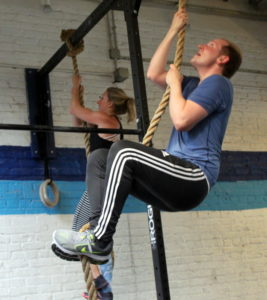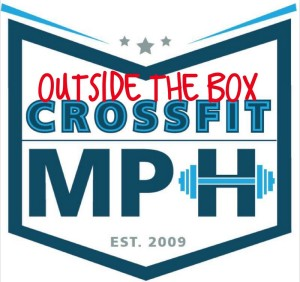## Tuesday, August 29, 2017

### Tuesday, August 29, 2017The Chief
five cycles, as many rounds as possible in three minutes:
3x power clean @ 135/95-lbs.
6x pushup
9x squat

A one minute break is given before repeating each cycle. The clock does not reset or stop between rounds.

This workout is scored by the number of rounds completed from all five cycles.

Compare results to May 22, 2012.

###### workout courtesy of crossfit.comfive cycles, as many rounds as possible in three minutes:
3x standing long jump
6x pushup
9x squat

A one minute break is given before repeating each cycle. The clock does not reset or stop between rounds.

This workout is scored by the number of rounds completed from all five cycles.

Post score to comments.

### 1 Comment

1.–Scores–
Sean F.: 22 rounds + 3x pc
David G.: 15 rounds + 6x pu (115-lbs.)
Walt C.: 17 rounds (115-lbs.)
Luke B.: 19 rounds + 2x pc (95-lbs.)
David C.: 17 runds + 3x pc (105-lbs.)
Adrian A.: 16 rounds + 1x squat (95-lbs.; sub: knee pushup)
Jerry I.: 13 rounds + 3x pc (95-lbs.)
Todd C..: 17 rounds (65-lbs.)
Miguel N.: 18 rounds + 3x pu (65-lbs.)
Christopher J.: 15 rounds (65-lbs.)
Miranda B.: 11 rounds + 5x pu (75-lbs.; sub: knee pushup)
Charlie K.: 16 rounds + 3x pu (85-lbs.)
Diana G.: 15 rounds + 1x pc (55-lbs.; sub: pushup/knee pushup)
Lauren I.: 10 rounds (55-lbs.; sub: hang power clean, knee pushup)
Carly B.: 15 rounds + 5x pu (65-lbs.; sub: knee pushup)
Ralph B.: 18 rounds + 6x pu
Jason O.: 23 rounds + 3x pc
Greg F.: 14 rounds + 5x squat (95-lbs.)
Sean Q.: 21 rounds + 2x dl (185-lbs.; sub: deadlift)
Dan A.: 18 rounds + 3x pc (105-lbs.)
Alex Z.: 17 rounds + 6x squat
Allie R.: 17 rounds (85-lbs.; sub: knee pushup)
Drew P.: 15 rounds + 3x hpc (sub: hang power clean, 4 cycles)
Blaine P.: 15 rounds + 1x pc (135-lbs.)
Borja G.: 22 rounds + 2x pu (115-lbs.; sub: hang power clean)
Andrew Z.: 16 rounds
Hari P.: 21 rounds + 1x pc
Chase H.: 16 rounds + 7x squat (125-lbs.)
Neil A.: 12 round s+ 6x pu (95-lbs.; sub: muscle clean)
Charles B.: 20 rounds (95-lbs.)
Alana L.: 16 rounds (75-lbs.)
Anna G.: 15 rounds + 3x pc (65-lbs.; sub: hang power clean)
Collin S.: 18 rounds (55-lbs.; sub: hang power clean)
Chelsea T.: 15 rounds + 1x pc (45-lbs.)
Dave R.: 22 rounds
Edgar O.: 15 rounds (40-lb. dbs; sub: db pushup)
Katherine C.: 12 rounds + 1x pu (45-lbs.; sub: knee pushup)
Cassie S.: 16 rounds + 3x pu (85-lbs.)
Matt W.: 21 rounds + 3x pc
Brad G.: 24 rounds+ 3x pc (95-lbs.)
Travers O.: 16 rounds + 6x pu (85-lbs.)
Ralph A.: 13 rounds + 1x pc (115-lbs.)
Michael H.: 19 rounds + 1x pc
Jamil W.: 16 rounds + 3x squat(75-lbs., sub: hang power clean)
Donal O.: 20 rounds + 1x pc
Brian K.: 10 + 1x pc
Elise C.: 15 rounds + 3x pu
Tara S.: 16 rounds + 4x pu
Christian M.: 20 rounds + 3x pc (115-lbs.; sub: prom squat)
Josh M.: 20 rounds+ 6x pu
Jason S.: 18 rounds + 2x pc (115-lbs.)
Stephen K.: 18 rounds + 3x pc (105-lbs.)
Chris C.: 28 rounds + 1x squat
David Sc.: 15 rounds (115-lbs.)
Candida M.: 21 rounds + 1x pc (65-lbs.)
Thomas R.: 18 rounds (115-lbs.)
Yordano L.: 15 rounds (85-lbs.)
Matt S.: 25 rounds + 3x pc (115-lbs.; sub: prom squat)
Jonathan H.: 15 rounds + 6x pu (115-lbs.)
Liz L.: 20 rounds + 3x squat (75-lbs.; sub: pushup x 3 cycles, knee pushup, 2 cycles)
Joe P.: 18 rounds + 1x pc
Bill G.: 27 rounds+ 2x pc (115-lbs.)
John P.: 22 rounds+ 6x squat
Mark Co.: 21 rounds
Sean Mc.: 18 rounds + 1x pc
Mark Ab.: 19 rounds + 3x squat
Francisco L.: 17 rounds + 6x pu (125-lbs.)
Patrick J.: 18 rounds (115-lbs.)
Kyle T.: 16 rounds + 2x pu (95-lbs.)
Carl L.: 19 rounds+ 1x pc (95-lbs.)
Adrienne V.: 15 rounds + 3x pu (85-lbs.; sub: pushup/knee pushup)
Rachel B.: 17 rounds + 1x pu (85-lbs.)
Jin D.: 14 rounds + 3x pc (75-lbs.)
Seth B.: 17 rounds + 3x pp (40-lbs. dc; sub: push press)